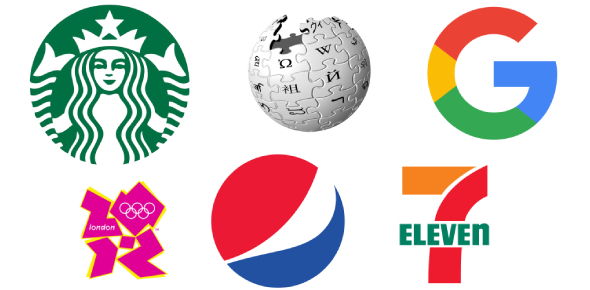# Introduction To Computer Science Test

23 Questions | Attempts: 102
ShareSettings• 1.
Consider the following code: Dim n as Integer = -8 n = 100 / n + 4 What is the value of n after the code is run?
• A.

-8.5

• B.

16.5

• C.

-8

• D.

16

• E.

-25

• 2.
Consider the following code: Dim n as Integer = -8 n = 100 / (n + 4) What is the value of n after the code is run?
• A.

-8

• B.

-8.5

• C.

14

• D.

14.5

• E.

-25

• 3.
Which of the following expressions might be used in a calculation to determine leap year?
• A.

Year - 2000

• B.

Year / 2000

• C.

Year / 4

• D.

Year Mod 4

• E.

Year \ 4

• 4.
What will be the value of z as a result of the following statement? Dim z as Integer z = 50 \ 15
• A.

3

• B.

5

• C.

3.6

• D.

750

• 5.
Assuming the variables x1, x2, y1, and y2 have been declared as integers, have been assigned values, and represent the coordinates of two points on the coordinate grid, write the statement to calculate the slope. Extra credit: Find the y intercept (e.g., the 'b' in y = mx + b)
• 6.
Declare four variables (String, boolean, integer, and double) that you would need if you were to write  Power School
• 7.
A label control is used to perform an action when clicked
• A.

True

• B.

False

• 8.
The most important information on a form should be in the upper-left corner of the form.
• A.

True

• B.

False

• 9.
The use of multiple fonts and multiple font sizes is a good idea.
• A.

True

• B.

False

• 10.
The arithmetic operators *, /, + and - all have the same level of precedence.
• A.

True

• B.

False

• 11.
You specify a default button by setting the form’s AcceptButton property to the name of the button.
• A.

True

• B.

False

• 12.
Whish property controls the caption displayed on the Forms title bar?
• A.

Text

• B.

TitleBar

• C.

Name

• D.

Caption

• 13.
The tool that displays text which the user is not allowed to edit while the application is running is _____.
• A.

Label

• B.

Text Box

• C.

• D.

Button

• 14.
TOE stands for
• A.

Text, Object, Environment

• B.

• C.

• D.

• 15.
A variable that can only be true or false is what kind?
• 16.
The name of a Visual Basic .NET variable must begin with a(n) _______.
• A.

Letter

• B.

Digit

• C.

Hyphen

• D.

Underscore

• 17.
The equals sign (=) is called the ________________ operator
• A.

Equal

• B.

Assignment

• C.

Validation

• D.

Evaluation

• 18.
Memory locations where programs store data are called:
• 19.
Red/green color blindness is common. When considering using those colors you should keep what in mind?
• A.

Red and green have specific meanings

• B.

Shape, position, or texture should also be used

• C.

Contrasting colors can often highlight each other

• D.

All of the above

• 20.
If I have a button that adds tax to a product price, what would be the most appropriate caption?
• A.

• B.

• C.

• D.

• 21.
Consider the following code sum = sum + Convert.toString(TextBox.Text) The variable sum is called a
• A.

Counter

• B.

Property

• C.

Accumulator

• D.

Parameter

• 22.
If I want to combing the contents of two text boxes into one label, which statememt would I use?
• A.

TextBox1.Text + TextBox2.text = Label1.text

• B.

Label1.text = TextBox1.Text + TextBox2.text

• C.

Label1 = TextBox1 + TextBox2

• D.

Label1.text(TextBox.Text1, TextBox2.text)

• 23.
Complete the following code by computing the total cost of purchasing show tickets that includes the service charge per ticket and tax Dim n as Innteger = Convert,toString(txtNumberofTickets.Text) Dim c as Double = Convert.toDouble(txtCostperTickket.Text) Dim service as Double = 2.75 ' Added on to each ticket Dim salesTax as Double = 0.075 lblTotalCost.text = ' Write the appripopriate expressionin the answer block

## Related TopicsBack to top
×

Wait!
Here's an interesting quiz for you.